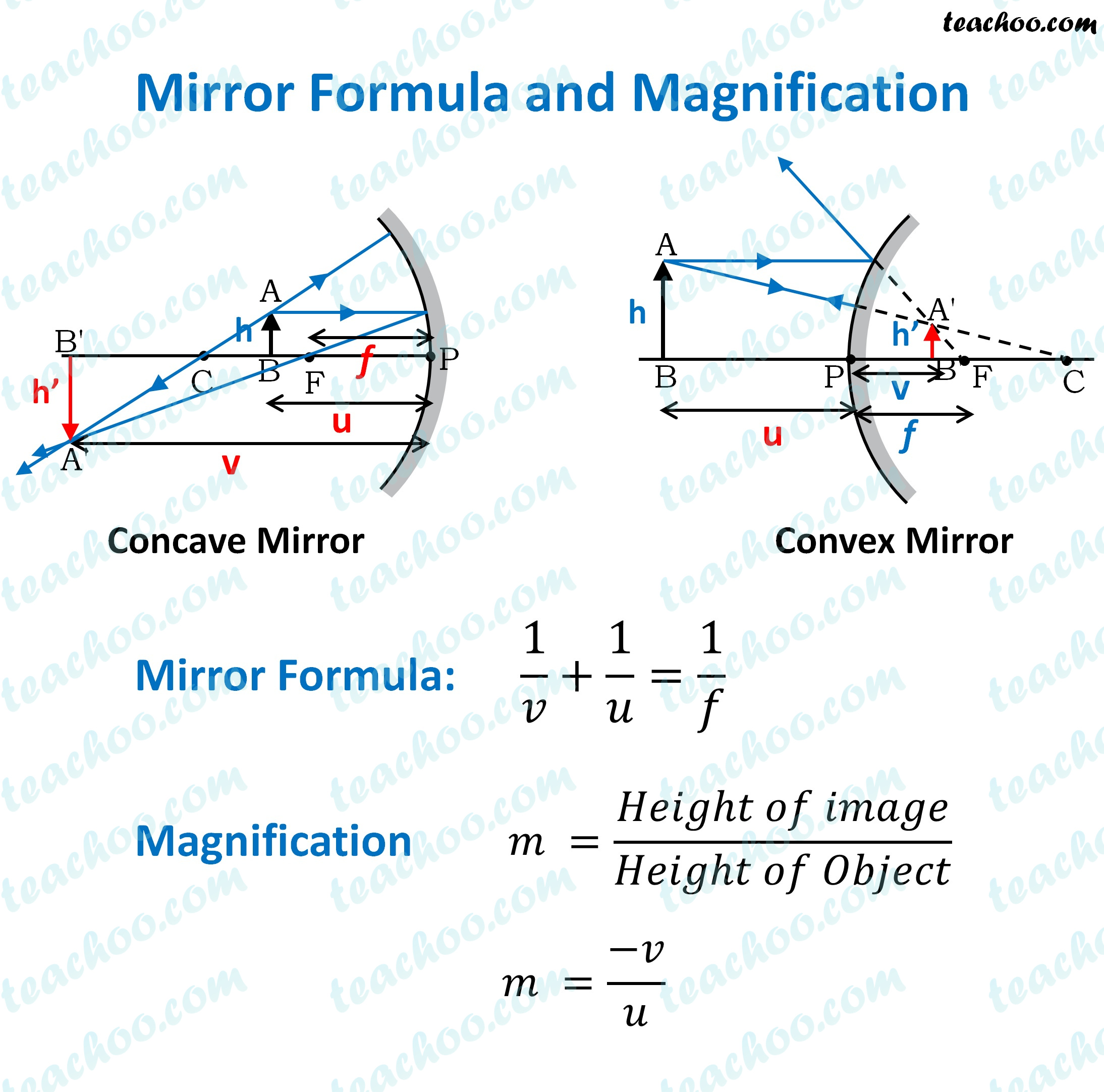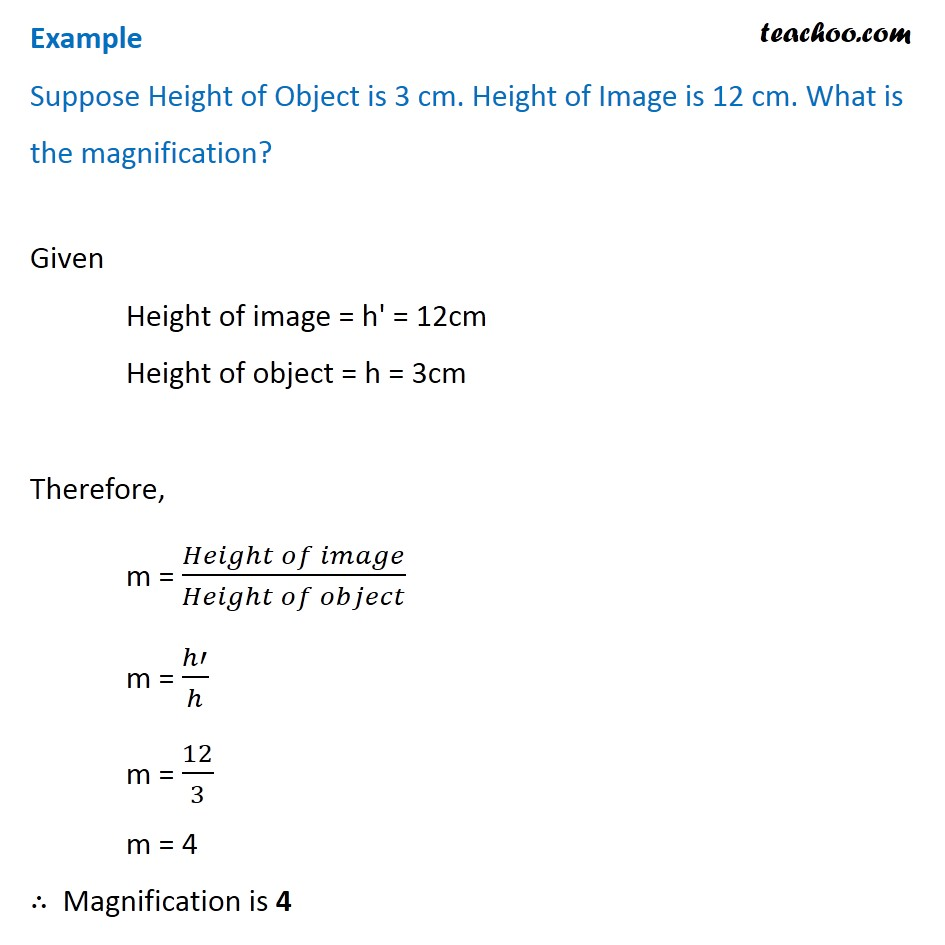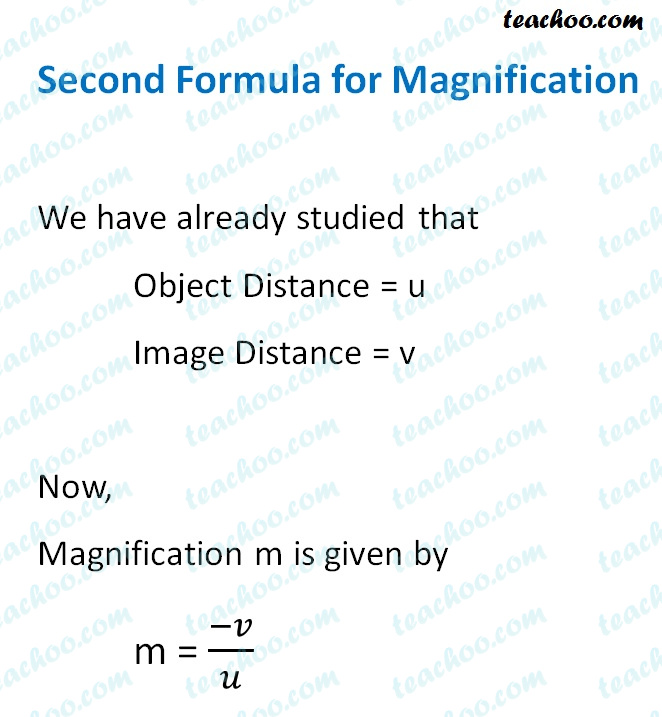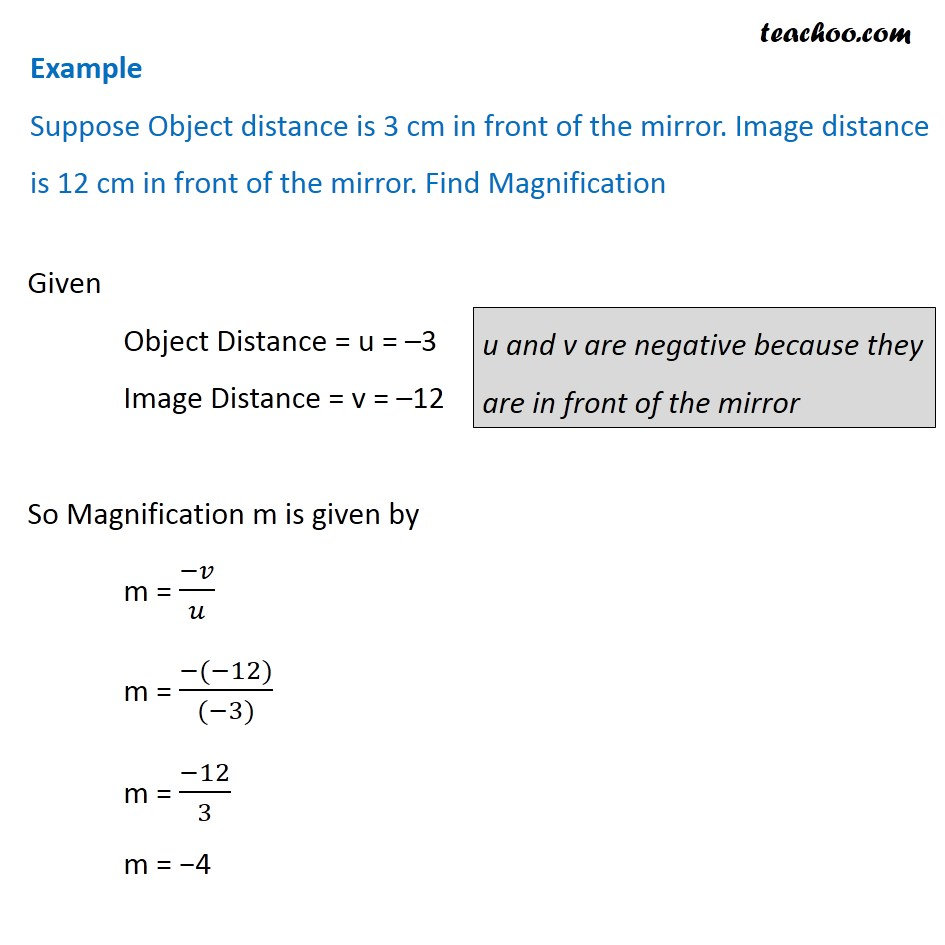Concepts

Class 10
Chapter 10 Class 10 - Light - Reflection and Refraction

Before we look at Mirror Formula, lets look at the basics

## What is Object Distance

Distance of Object from Pole is called Object Distance

It is denoted by u

## What is Image Distance

Distance of Image formed from Pole of Mirror is called Image Distance

It is denoted by v

## What is Focal length

The Distance between focus and the Pole is Called Focal Length

It is denoted by f

## What is Mirror Formula

This formula denotes relationship between

• Object Distance(u)
• Image Distance(v)
• Focal length(f)Note : - We follow the sign convention for mirrors
In the image, the red text is negative, and blue text is positive

Thus,

• If Virtual image is formed, v is positive
• If Real Image is formed, v is negative
• Object Distance (u) is always negative as it is on left side of Mirror
• Focal Length of Concave Mirror is Negative. It is because Focus is in front of mirror
• Focal Length of Convex Mirror is Positive. It is because Focus is behind mirror

In addition to the mirror formula,

we have the magnification formula

## What is the magnification formula?

It is Ratio of Height of Image to the Height of Object

Suppose Height of object is h

Height of Image is h'

Magnification is m

So

Magnification = Height of Image/ Height of Object

m = h'/h

Let's do a few examples## Second Formula for Magnification

There is another formula of magnificationNote : -   If magnification (m) is positive , It means image formed is virtual and erect
If magnification (m) is negative, It means image formed is real and inverted## Questions

Example 10.1 - A convex mirror used for rear-view on an automobile has a radius of curvature of 3.00 m. If a bus is located at 5.00 m from this mirror, find the position, nature and size of the image

Example 10.2 - An object, 4.0 cm in size, is placed at 25.0 cm in front of a concave mirror of focal length 15.0 cm. At what distance from the mirror should a screen be placed in order to obtain a sharp image? Find the nature and the size of the image.

Question 1 Page 171 - Find the focal length of a convex mirror whose radius of curvature is 32 cm

Question 2 Page 171 - A concave mirror produces three times magnified (enlarged) real image an object placed at 10 cm in front of it. Where is the image located?

NCERT Question 7 - We wish to obtain an erect image of an object, using a concave mirror of focal length 15 cm. What should be the range of distance of the object from the mirror? What is the nature of the image? Is the image larger or smaller than the object? Draw a ray diagram to show the image formation in this case.

NCERT Question 12 - An object is placed at a distance of 10 cm from a convex mirror of focal length 15 cm. Find the position and nature of the image

NCERT Question 13 - The magnification produced by a plane mirror is +1. What does this mean?

NCERT Question 14 - An object 5.0 cm in length is placed at a distance of 20 cm in front of a convex mirror of radius of curvature 30 cm. Find the position of the image, its nature and size.

NCERT Question 15 - An object of size 7.0 cm is placed at 27 cm in front of a concave mirror of focal length 18 cm. At what distance from the mirror should a screen be placed, so that a sharp focussed image can be obtained? Find the size and the nature of the image.

Learn in your speed, with individual attention - Teachoo Maths 1-on-1 Class

### Transcript

Example Suppose Height of Object is 3 cm. Height of Image is 12 cm. What is the magnification? Given Height of image = h' = 12cm Height of object = h = 3cm Therefore, m = (𝐻𝑒𝑖𝑔ℎ𝑡 𝑜𝑓 𝑖𝑚𝑎𝑔𝑒)/(𝐻𝑒𝑖𝑔ℎ𝑡 𝑜𝑓 𝑜𝑏𝑗𝑒𝑐𝑡) m = ℎ′/ℎ m = 12/3 m = 4 ∴ Magnification is 4 Second Formula for Magnification We have already studied that Object Distance = u Image Distance = v Now, Magnification m is given by m = (−𝑣)/𝑢 Example Suppose Object distance is 3 cm in front of the mirror. Image distance is 12 cm in front of the mirror. Find Magnification Given Object Distance = u = –3 Image Distance = v = –12 So Magnification m is given by m = (−𝑣)/𝑢 m = (−(−12))/((−3)) m = (−12)/3 m = −4 u and v are negative because they are in front of the mirror